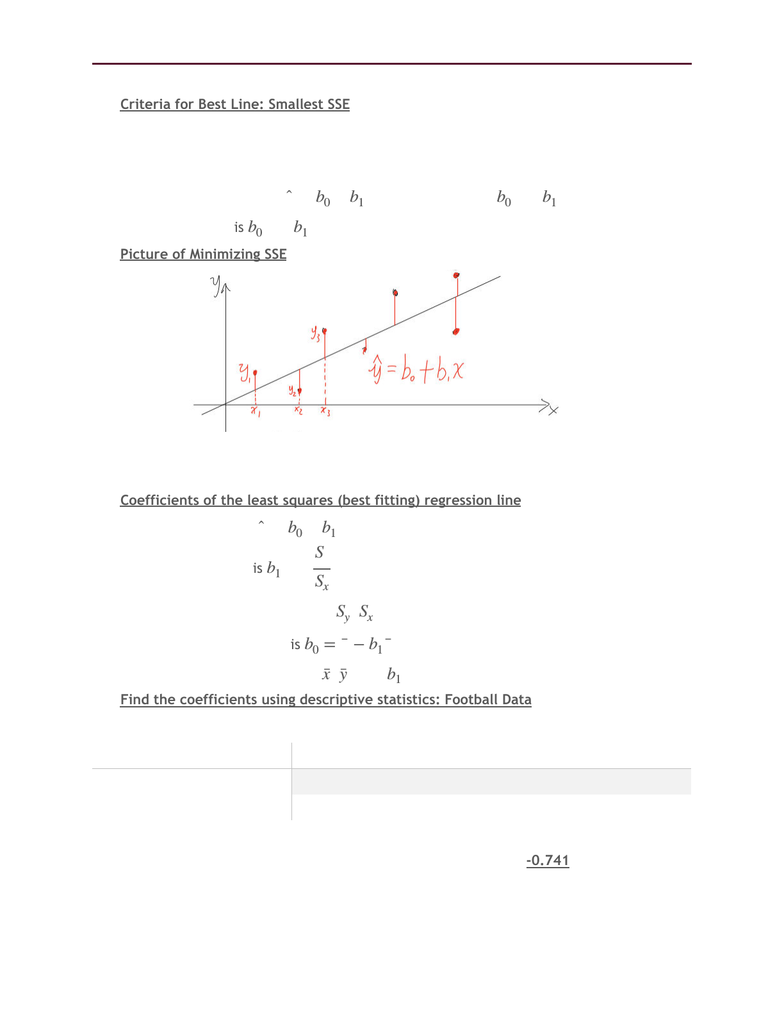# STAT 1430 Lecture Notes - Lecture 6: Pearson Product-Moment Correlation Coefficient, Descriptive Statistics, Regression Analysis

197 views4 pagesSTAT1430.01–Lecture6–Correlation and Regression Jan 24, 2019
Criteria for Best Line: Smallest SSE
oSSE= Sum of the square for error
oFind the line with the smallest SSE, from all the potential lines that go through
the data.
oIn the equation , find the values of and that minimize SSE.
oFrom this and you create the “least squares regression line.
Picture of Minimizing SSE
o
oMany Possible Lines
oTake the one with the smallest (“least”) SSE
Coefficients of the least squares (best fitting) regression line
oBest line is
oBest slope is = r *
Here we need r, ,
oBest y-intercept is
Here we need , after is computed, only to 5 numbers find best fit
Find the coefficients using descriptive statistics: Football Data
oDescriptive statistics: Temperature, Coffees
oCorrelations: Temperature, Coffees
oPearson correlation of Temperature and Coffees = r = -0.741
oP-Value = 0.000
̂
y=b0+b1x
b0
b1
b0
b1
̂
y=b0+b1x
b1
Sy
Sx
Sy
Sx
¯x
¯y
b1
Variable
Mean
Standard Deviation
Te m p e r a t u r e
35.08
16.29
Coffees
29913
12174
Unlock document

This preview shows page 1 of the document.
Unlock all 4 pages and 3 million more documents.

## Document Summary

Criteria for best line: smallest sse: sse= sum of the square for error, find the line with the smallest sse, from all the potential lines that go through the data, in the equation. , find the values of b0 and b1 that minimize sse: from this b0 and b1 you create the least squares regression line. Picture of minimizing sse: many possible lines, take the one with the smallest ( least ) sse. Coefficients of the least squares (best fitting) regression line: best line is. Y = b0 + b1x: best slope is = r * b1. Sy sx: here we need r, , best y-intercept is b0 = y b1 x, here we need , after. X y b1 is computed, only to 5 numbers find best fit. Find the coefficients using descriptive statistics: football data: descriptive statistics: temperature, coffees. 12174: correlations: temperature, coffees, pearson correlation of temperature and coffees = r = -0. 741, p-value = 0. 000.

## Get access

\$10 USD/m
Billed \$120 USD annuallyHomework Help
Study Guides
Textbook Solutions
Class Notes
Textbook Notes
Booster Class